U.S. Department of Transportation
1200 New Jersey Avenue, SE
Washington, DC 20590
202-366-4000

Federal Highway Administration Research and Technology
Coordinating, Developing, and Delivering Highway Transportation InnovationsThis report is an archived publication and may contain dated technical, contact, and link information
 Federal Highway Administration > Publications > Research Publications > 98133 > Ch05_04.Cfm > Accident Models for Two-Lane Rural Roads: Segment and Intersections
Publication Number: FHWA-RD-98-133
Date: October 1998

# Accident Models for Two-Lane Rural Roads: Segment and Intersections

## 5. Modeling

Logistic Modeling

Logistic modeling was done in this study on the Minnesota data to determine whether the probability of a serious accident given that an accident has occurred can be related to highway and intersection variables. The variable INJACC counts the number of injury accidents (i.e., other than property damage only accidents) and includes accidents with non-incapacitating injuries and possible injuries, whereas the focus of the logistic modeling is serious accidents (fatal or injury accidents). All sites with zero accidents were excluded.

Although the results are inconclusive, we present them here since the methodology may be of interest.

Theory

Logistic regression is used to estimate probabilities for binary data or discrete ordinal data. In our case two severity classes are used: serious accidents and other accidents. The probability of an accident being severe is represented as a function of highway and intersection variables of generalized linear type, typically a logistic function of a linear combination of these variables.

A variable Y for each accident is defined as follows: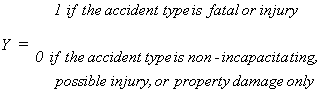Then P1 is the probability that Y has the value 1 given the value x = (x1,...,xk) of the highway characteristics at the accident site . With the logistic function, the model takes the form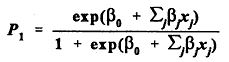This functional form guarantees that P1 will always be a number between 0 and 1. Since P1 is the probability that an accident is severe (Y = 1) given the values of x, then 1 - P1 is the probability that an accident is not severe (Y = 0). The likelihood function for all the observed severities, derived from the binomial distribution under the assumption that the accidents are independent events, is: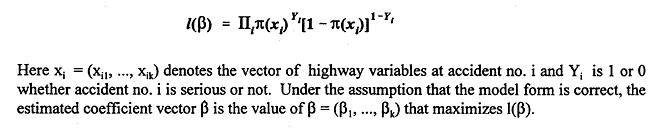A measure of goodness of fit used on this model is the rank correlation (available in the SAS procedure LOGISTIC). All possible accident pairs with distinct severities are formed from the data, and then one calculates:

total = t = the total number of pairs

concordance = nc = the number of pairs for which the model predicts higher probability of a severe accident for the member of the pair that had the more severe accident

discordance = nd = the number of pairs for which the model predicts higher probability of a severe accident for the member of the pair that had the less severe accident

ties = t - nc - nd = the number of pairs with same predicted probability of a severe accident .

Probabilities are grouped into intervals of length .02 and are considered equal if they lie in the same interval. Finally one calculates

c = (nc + 0.5(t - nc - nd))/t.

The statistic c takes values between 0 and 1, and achieves the value .5 on average if a member of each pair is chosen with equal probability. Thus the farther above .5 c is the better the model.

Results

On the 619 Minnesota segments of this study in the time period 1985-89 there were a total of 1,694 accidents, 121 of them serious. The models that result from maximum likelihood techniques showed no significant variables other than commercial ADT percentage T. Horizontal alignment or vertical alignment, but not both, had positive coefficients but the P-values were insignificant (one form of horizontal, not shown here, had a P-value of .306). One typical run yielded equation (5.18):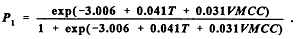(5.18)

The P-values and statistic c are shown below.

Table 38. Logistic Model for Serious Accident Probability, Minnesota Segments

 PARAMETER ESTIMATE P-value Intercept -3.0060 0.0001 Percent of commercial vehicles = T 0.0413 0.0310 Crests of Type I rate = VMCC 0.0314 0.5634 Concordance = 53.1%, Discordance = 41.6%, c = 55.8%

The statistic c differs from 50% by an appreciable but modest amount.

For the three-legged Minnesota intersections, from 1985 to 1989, there were 524 accidents, 34 of them serious. Accident severity does not seem to be significantly affected by the value of the Conflict Index CINDEX. However, as equation (5.19) shows, horizontal alignment (out to 764 feet in each direction) tends to increase the severity, while severity is negatively influenced by vertical alignment (The variable VCEI is a variant of VCI, going out to 764 feet rather than 250 feet). Since there are very few serious accidents, this result contrary to expectation may reflect peculiarities in the sample.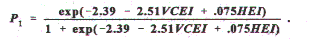(5.19)

Table 39. Logistic Model for Serious Accident Probability, MN 3-Legged Intersections

 PARAMETER ESTIMATE P-value Intercept -2.39 0.0001 Crest curve rate VCEI (out to " 764') -2.5099 0.03 Horizontal curvature rate HEI (out to " 764') 0.0753 0.09 Concordance = 60.4%, Discordance = 33.5%, c = 63.4%

For the four-legged Minnesota intersections, from 1985 to 1989, there were 494 accidents, 58 of them serious. The model below was developed.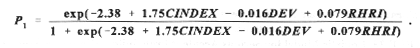Alignments were not at all significant. Instead the conflict index and the angular deviation from 90º were marginally so. Roadside Hazard Rating, although not significant, was also retained.

Table 40. Logistic Model for Serious Accident Probability, MN 4-Legged Intersections

 PARAMETER ESTIMATE P-value Intercept -2.38 0.0001 Conflict index CINDEX 1.75 0.10 Angle DEV -0.016 0.20 Roadside Hazard Rating RHRI 0.079 0.55 Concordance = 57.1%, Discordance = 40.3%, c = 58.4%